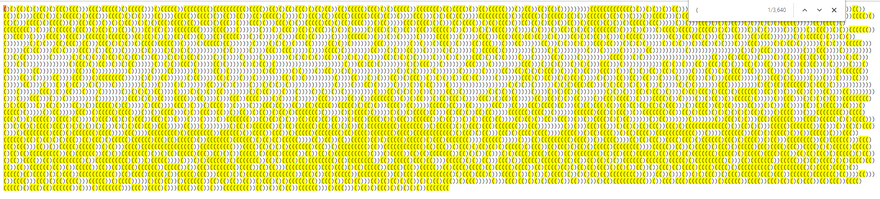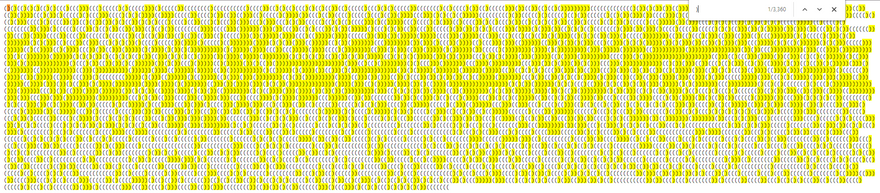## CodeNewbie Community 🌱Xavier Jouvenot

Posted on • Originally published at 10xlearner.com

# Advent Of Code - Not Quite Lisp - Puzzle 1

Hello there ! I'm Xavier Jouvenot and here is the first part of a long series on Advent Of Code.

For this first post, we will start with the problem from the 1st December 2015, named "Not Quite Lisp".
The solution I will propose in c++, but the reasoning can be applied to other languages.

## The Problem

The full version of this problem can be found directly on the Advent of Code website, I will only describe the essence of the problem here:

Santa needs to find the floor on which he will leave his presents, but he only has instructions with `(` and `)` which respectively indicate that he should go up one floor or go down one floor.
Here are some examples:

• `(((` results in floor `3`
• `)))` results in floor `-3`
• `(()))(` results in floor `0`

### No Code Solution

First of all, why write a program, when you avoid doing so.
Let's analyze the problem a little.

We want to know the final floor, the one on which Santa will be in the end. And that is the result of all the going up one floor and going down one floor. The result can then be described as `final Santa floor = (all the time Santa goes up) - (all the time Santa goes down)`.

So if we know how many times Santa goes up, or how many `(` there are, and down, or how many `)` there are, we won. And for that just using `CTRL-F` is enough to get the number of occurrences of `(` and `)`.And voilà. Here you can have the solution by subtracting the 2 number of parenthesis occurrences without writing even a line of code by yourself, since somebody has written the `CTRL-F` functionalities 😉.

### C++ Solution

Even if we can find the solution without coding, we can do it, for the exercise.

To find the solution, we can use some naive approach by iterating on the input while tracking the floor we are in, to ultimately arrive at the solution. But, we can use the same approach we used in the no-code solution, by counting the number of occurrences for each parenthesis.

``````PuzzleSolution computeSolution(const std::string_view input) {
const auto openParenthesisCount =
std::count(std::begin(input), std::end(input), '(');
const auto closeParenthesisCount =
std::count(std::begin(input), std::end(input), ')');

return openParenthesisCount - closeParenthesisCount;
}
``````

Simple, but efficient 😃.

This is not the only solution of course. Two other solutions I have in mind are the naive approach and the recursive one that I won't describe here.

## Part 2

After completing the first part of this puzzle, surprise !! (at least for me it was), you unlocked the second part.

This part consists of finding the position of the first character that makes Santa go into the basement.
Unlike the Part 1, there is no simple and quick tricks without some programming.
So here we go.

### Solution

Once again, we can use the std to find the solution to our problem.

``````PuzzleSolution computeSolution(const std::string_view input) {
auto floor{0};
const auto firstEnteringInTheBasement = std::find_if(
std::begin(input), std::end(input), [&floor](const auto &character) {
if (character == '(') {
++floor;
} else if (character == ')') {
--floor;
}
return floor < 0;
});
assert(firstEnteringInTheBasement != std::end(input));

return std::distance(std::begin(input), firstEnteringInTheBasement) + 1;
}
``````

And that's basically all we need to solve this problem.

## Conclusion

You can note that the solutions written in this post, don't include all the sources to make running programs, but only the interesting part of the sources to solve this problem.
If you want to see the programs from end to end, you can go on my GitHub account, explore the full solution, add comments or ask questions if you want to.

Here is the list of std method that we have used, I can't encourage you enough to look at their definitions :

Thanks for you reading, hope you liked it 😃
And until the next part, have fun learning and growing.